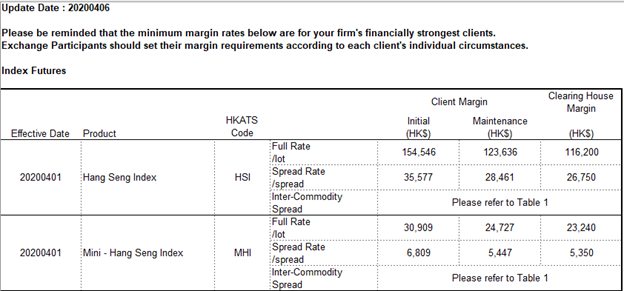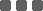## Overview of Leverage and Margin

#### Economy & Market

Most of the times, the terms leverage and margin are used together. Although they both involve borrowing, leverage and margin are in fact interconnected but different thing.

### What is Leverage?

Leverage refers to using borrowed capital as a funding source for investment to increase the potential return. In Corporate Finance, leverage can also refer to the amount of debt a firm uses to finance assets. That is, instead of issuing stock to raise capital, companies can use debt financing to invest in business operations in an attempt to increase shareholder value.

When a company or investment is said to be "highly leveraged", it means that it has more debt than equity. A common way to measure leverage is using Leverage Ratio, which is defined as:

Leverage Ratio = Asset/Equity = 1 + Debt/Equity

As seen from the formula, the value of leverage ratio is floored at 1 when there involves no borrowing, and has no upper bound theoretically. For example, suppose a bank has asset of US100 billion, where US10 billion come from Share holders and US90 billion come from depositors, then the bank will carry a leverage ratio of 10.

### What is Margin?

Margin is a way to create leverage!

In securities trading, a margin account allows you to borrow money from a broker at a fixed interest rate to purchase securities in the anticipation of receiving considerably higher returns. In practice, it includes buying an asset where the buyer pays only a percentage of the asset's value and borrows the rest from the bank or broker. The broker acts as a lender and the securities in the investor's account act as collateral.

Using margin to purchase securities is effectively like using the current cash or securities already in your account as collateral for a loan. The collateralized loan comes with an interest rate that must be paid. The investor is in fact taking leverage with the borrowed money, and therefore both the losses and gains will be magnified.

When money is borrowed from a brokerage firm to invest in other financial instruments, margin will be the difference between the total value of securities held in an investor's account and the loan amount from the broker. In other words, a margin refers to the amount of equity an investor has in their brokerage account. i.e.

Margin Amount = (Account Asset Value) - (Borrowed Amount) = Equity held in broker account

In reality, there are two common quotations for margin requirement.

1. Fixed margin amount
2. This quotation approach is mainly adopted by many Exchanges for the advantages of fast computation and the convenience for cash transfers.

For example, at the time of writing, HKEx (https://www.hkex.com.hk/Services/Clearing/Listed-Derivatives/Risk-Management/Margin/Margin-Tables?sc_lang=en) require investors to pay HK\$154,546 as margin in order to purchase 1 lot of HSI Index Future.3. Margin as a Percentage
4. In contrast to Fixed Margin approach, Margin as a Percentage would be more appropriate for risk management to deal with changing security price level. This approach is also widely adopted by other OTC Brokers such as Crypto and FX brokers.

As an example, if your margin account is subject to an initial margin requirement of 60%, and you purchase \$1,000,000 worth of stocks, then your margin would be \$600,000, where the rest would be borrowed from the broker.

### How Margin is related to Leverage?

Following the examples above,

1. As HSI Index Future has a price magnifier of 50, meaning that 1 index point change will lead to ±HK\$50. Suppose current HSI Index Future price is 25000, and buying 1 lot of HSI Index Future require HK\$154,546 as margin. Then, leverage ratio is calculated to be (\$25000*50)/\$154,546 = 8.0882

2. You pay \$600,000 to get stocks worth \$1,000,000. Thus, leverage ratio is calculated to be \$1,000,000/\$600,000 = 1.6667.

As you can imagine, the lower is the margin requirement, the higher is the leverage ratio. In general,

Leverage Ratio = 1/(Margin Requirement)

### How Margin and Leverage involved in P/L Calculation?

Once the leverage ratio is derived, the PL figure can simply be computed as

PLk,t = Vk,t × (Pk,t - Pk,t-1) × Lk - ck

where

• k is the k-th security in the account portfolio
• PLk,t is the daily profit/loss at day t
• Pk,t is the closing price at day t
• Vk,t is the outstanding number of share/ volume/ inventory at day t
• Lk is the leverage ratio for the k-th security in the margin account
• ck is the cost of carrying the k-th security in the margin account (eg. interest, tax, roll-over, etc)

The formula showed that leverage acts as a multiplicative factor, and that's why taking leverage could scale up potential profit or loss.

For leveraged trading where traders can take out a loan based on the amount of cash held in their brokerage account, buying power refers to the amount of money available for investors to purchase securities. Mathematically,

Buying Power = (Leverage Ratio)×(Investor's equity)

For example, suppose an investor made an initial deposit US\$100,000 to a broker 20:1 leverage account. Then, the investor would be able to purchase, at maximum, US\$2,000,000 worth of securities. Hence, the investor would have a buying power of US\$2,000,000 for this broker account.

### Conclusion

Leverage is a double-edged sword which can magnify both gains and losses. If an investor uses leverage to make an investment and the investment moves against the investor, his/her loss will be much greater than it would have been if he/she had not leveraged the investment. In reality, you should better consider your financial situation, investment objectives, experience, and risk tolerance, before making any leveraged investment decision.

### Categories

Hot Topic

24 0 1 2023-02-03

Request for parameter optimization function

70 1 1 2023-01-21

187 4 6 2023-01-12

Guideline to call App function in strategy script

155 0 5 2023-01-04

100 2 0 2023-01-03

Guideline to setup OTP Authenticator

143 0 0 2022-12-26

The Opportunities of a Grid Trading System

271 0 1 2022-12-18

3 Common Order Types You Should Know

160 1 2 2022-12-13

Guide to publish your app on App Store

234 4 6 2022-12-02